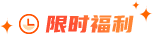### Python语言如何解析参数？

每种编程语言都有创建脚本并从终端运行它们或被其他程序调用的功能，在运行此类脚本时，我们经常需要传递脚本所需的参数，以便在脚本内执行各种功能，那你知道Python语言如何解析参数？请看下文：Python解析参数的三种方法有哪些？

第一种方法是使用argparse，它是一个流行的Python模块，专门用于命令行解析；另一种方法是读取JSON文件，我们可以在其中放置所有超参数，第三种方法也是鲜为人知的方式，也就是使用YAML文件。

1、使用argparse

首先，我们可以创建一个文件 train.py，在其中我们有导入数据、在训练数据上训练模型并在测试集上对其进行评估的基本程序：

import pandas as pd

import numpy as np

from sklearn.ensemble import RandomForestRegressor

from sklearn.model_selection import train_test_split

from sklearn.preprocessing import StandardScaler

from sklearn.metrics import mean_squared_error, mean_absolute_error

from options import train_options

opt = train_options()

X=df.drop(['instant','dteday','atemp','casual','registered','cnt'],axis=1).values

y =df['cnt'].values

X_train, X_test, y_train, y_test = train_test_split(X, y, test_size=0.2)

if opt.normalize == True:

scaler = StandardScaler()

X = scaler.fit_transform(X)

rf = RandomForestRegressor(n_estimators=opt.n_estimators,max_features=opt.max_features,max_depth=opt.max_depth)

model = rf.fit(X_train,y_train)

y_pred = model.predict(X_test)

rmse = np.sqrt(mean_squared_error(y_pred, y_test))

mae = mean_absolute_error(y_pred, y_test)

print("rmse: ",rmse)

print("mae: ",mae)

在代码中，我们还导入了包含在 options.py 文件中的 train_options 函数。后一个文件是一个 Python 文件，我们可以从中更改 train.py 中考虑的超参数：

import argparse

def train_options():

parser = argparse.ArgumentParser()

parser.add_argument("--n_estimators", default=100, type=int, help='number of estimators')

parser.add_argument("--max_features", default=6, type=int, help='maximum of features',)

opt = parser.parse_args()

return opt

2、使用JSON文件

和前面一样，我们可以保持类似的文件结构。在这种情况下，我们将 options.py 文件替换为 JSON 文件。换句话说，我们想在 JSON 文件中指定超参数的值并将它们传递给 train.py 文件。与 argparse 库相比，JSON 文件可以是一种快速且直观的替代方案，它利用键值对来存储数据。下面我们创建一个 options.json 文件，其中包含我们稍后需要传递给其他代码的数据。

{

"normalize":true,

"n_estimators":100,

"max_features":6,

"max_depth":5

}

如上所见，它与 Python 字典非常相似。但是与字典不同的是，它包含文本/字符串格式的数据。此外，还有一些语法略有不同的常见数据类型。例如，布尔值是 false/true，而 Python 识别 False/True。JSON 中其他可能的值是数组，它们用方括号表示为 Python 列表。

3、使用YAML文件

最后一种选择是利用 YAML 的潜力。与 JSON 文件一样，我们将 Python 代码中的 YAML 文件作为字典读取，以访问超参数的值。YAML 是一种人类可读的数据表示语言，其中层次结构使用双空格字符表示，而不是像 JSON 文件中的括号。下面我们展示 options.yaml 文件将包含的内容：

normalize: True

n_estimators: 100

max_features: 6

max_depth: 5

在 train.py 中，我们打开 options.yaml 文件，该文件将始终使用 load 方法转换为 Python 字典，这一次是从 yaml 库中导入的：

import yaml

f = open('options.yaml','rb')

和前面一样，我们可以使用字典所需的语法访问超参数的值。

注：本文部分文字和图片来源于网络，如有侵权，请联系删除。版权归原作者所有!此页面下方声明无效！### Python师资团队更多>>

#### 开班信息 北京校区 北京校区 大连校区 广州校区 成都校区 杭州校区 长沙校区 合肥校区 南京校区 上海校区 深圳校区 武汉校区 郑州校区 西安校区 青岛校区 重庆校区 太原校区 沈阳校区